Courses

# Partial Derivatives And Euler's Equation MCQ Level - 1

## 10 Questions MCQ Test Topic wise Tests for IIT JAM Physics | Partial Derivatives And Euler's Equation MCQ Level - 1

Description
This mock test of Partial Derivatives And Euler's Equation MCQ Level - 1 for Physics helps you for every Physics entrance exam. This contains 10 Multiple Choice Questions for Physics Partial Derivatives And Euler's Equation MCQ Level - 1 (mcq) to study with solutions a complete question bank. The solved questions answers in this Partial Derivatives And Euler's Equation MCQ Level - 1 quiz give you a good mix of easy questions and tough questions. Physics students definitely take this Partial Derivatives And Euler's Equation MCQ Level - 1 exercise for a better result in the exam. You can find other Partial Derivatives And Euler's Equation MCQ Level - 1 extra questions, long questions & short questions for Physics on EduRev as well by searching above.
QUESTION: 1

### If z = xy In (x, y) then

Solution: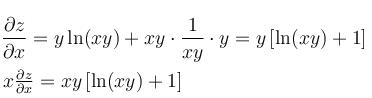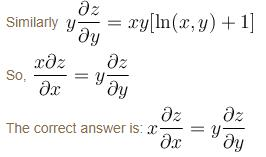QUESTION: 2

### Suppose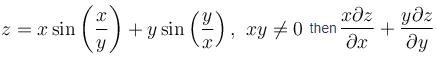is equal to

Solution: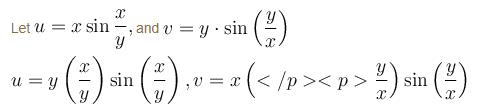u and v are homogeneous function of degree one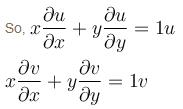on adding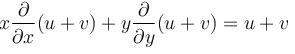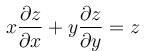QUESTION: 3

### If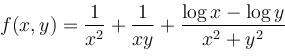then the value of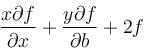Solution: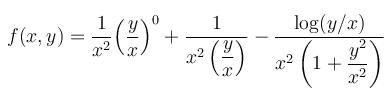f(xy) is homogeneous function of degree –2
So, using Euler's equation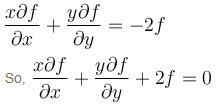QUESTION: 4

If  u = sin x/l*sin y/m * sin z/n * cospt  satisfy the equation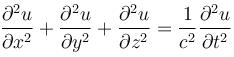then

Solution: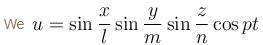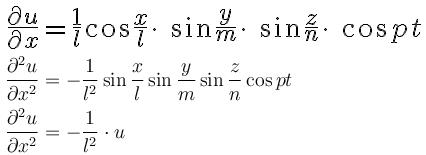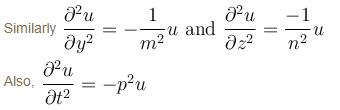Again using the given condition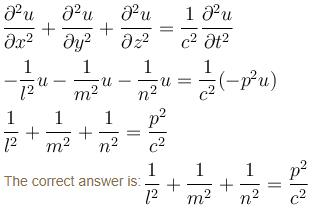QUESTION: 5

If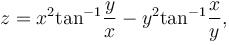then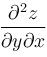equal to

Solution:

The correct answer is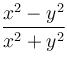QUESTION: 6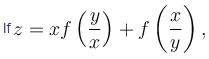then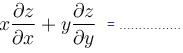Solution: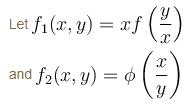f1 is homogeneous of degree 1 and f2 is homogeneous of degree zero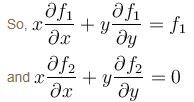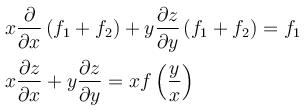The correct answers are: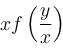QUESTION: 7

If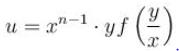then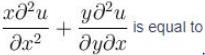Solution:

We have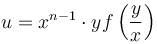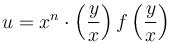u is homogeneous function of degree n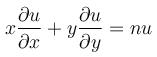Now differentiate partially w.r.t. x again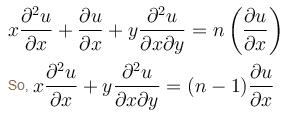The correct answer is: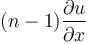QUESTION: 8

Find a function w = f(xy) whose first partial derivatives are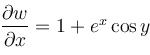and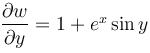and whose value at point (ln2, 0) is ln2.

Solution:

We σw/σx=1+excos y

Integrate both w.r.t.x

w(x,y)  =x+ex cosy+f(y)

σw/σy=0-exsiny+f’(y)

Given =-exsiny+2y

So,on comparing the above two equation

f’(y)=2y

f(y)=y2+c(on integration)

so, w(x,y)=x+excos y+y2+c

Now, using (In2,0)is In2,we get

C=-2

Hence, w(x,y)=x+y2+excosy-2

The correct answer is, option C; w=x+y2+excosy-2

QUESTION: 9

If u = sin-1 [(x3 + y3 + z3)/(ax + by + cz)] then xdu/dx + ydu/dy + zdu/dz equal to

Solution: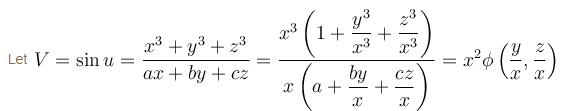So, by Euler's theorem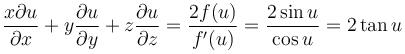The correct answer is: 2 tan u

QUESTION: 10

The contour on xy plane where partial derivative of x2 + y2 with respect to y is equal to the partial derivative of 6y + 4x w.r.t. x is

Solution:

So,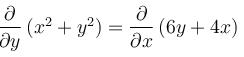2y = 4
y = 2
The correct answer is: y = 2